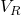## A 2μF capacitor holds a charge of 24μC. The capacitor is discharged through a 3Ω resistor. What is the voltage across the capacitor at the t

Question

A 2μF capacitor holds a charge of 24μC. The capacitor is discharged through a 3Ω resistor. What is the voltage across the capacitor at the time that the current through the resistor is 3A?

a. VC = 9V
b. VC = 8V
c. VC = 12V
d. VC = 3V

in progress 0
7 months 2021-07-29T20:26:43+00:00 2 Answers 3 views 0

The voltage drop across the capacitor is 3V

(d) VC = 3V

Explanation:

Given;

capacitance of the capacitor, C =  2μF

charge of the capacitor, Q =  24μC

resistance of the resistor, R = 3Ω

current through the resistor, I = 3 A

Initial voltage across the capacitor, V₀:

VC₀ = Q/C = 24μ / 2μ = 12 V

voltage across the resistor:= IR = 3 x 3 = 9 V

Voltage drop across the capacitor at the time that the current through the resistor is 3A:

VC = VC₀  –VC = 12V – 9V

VC = 3V

Therefore, the voltage drop across the capacitor is 3V

(d) VC = 3V

d. VC = 3V

Explanation:

If a 2μF capacitor holds a charge of 24μC, the voltage across the capacitor initially Vc is expressed as;

Vc = Q/C {since Q = CV}

Vc = 24μC/2μF

Vc = 12Volts

The voltage across the resistor is derived according to ohm’s law;

VR = IR

Given R = 3Ω and I = 3A

VR = 3×3

VR = 9V

If the capacitor is discharged through the resistor, the voltage across the capacitor at the time that the current through the resistor is 3A is expressed as;

VC = Vc – VR

Vc is the voltage across the capacitor during discharging.

VC = 12-9

VC = 3V